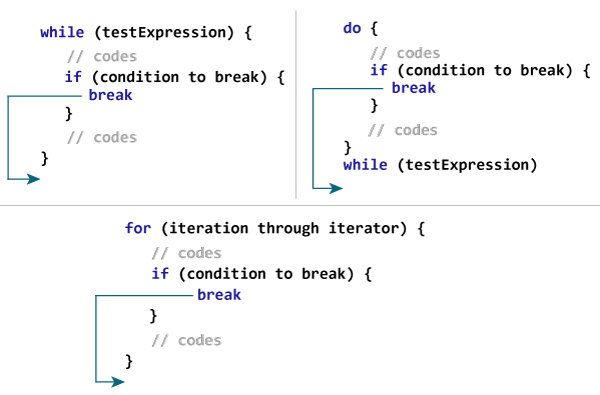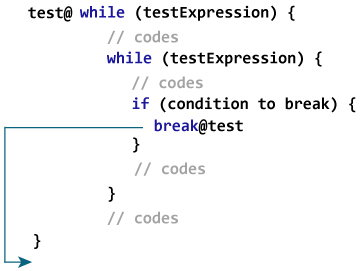Kotlin break Expression

# Kotlin break Expression

#### In this tutorial, you will learn to use break to terminate a loop. Also, you will also learn about break labels.

Suppose you are working with loops. It is sometimes desirable to terminate the loop immediately without checking the test expression.

In such case, `break` is used. It terminates the nearest enclosing loop when encountered (without checking the test expression). This is similar to how break statement works in Java.

## How break works?

It is almost always used with if..else construct. For example,

```for (...) {
if (testExpression) {
break
}
}```

If testExpression is evaluated to `true`, `break` is executed which terminates the `for` loop.### Example: Kotlin break

``````fun main(args: Array<String>) {

for (i in 1..10) {
if (i == 5) {
break
}
println(i)
}
}``````

When you run the program, the output will be:

```1
2
3
4
```

When the value of i is equal to 5, expression `i == 5` inside `if` is evaluated to `true`, and `break` is executed. This terminates the for loop.

### Example: Calculate Sum Until User enters 0

The program below calculates the sum of numbers entered by the user until user enters 0.

Visit Kotlin Basic Input Output to learn more on how to take input from the user.

``````fun main(args: Array<String>) {

var sum = 0
var number: Int

while (true) {
print("Enter a number: ")

if (number == 0)
break

sum += number
}

print("sum = \$sum")
}``````

When you run the program, the output will be:

```Enter a number: 4
Enter a number: 12
Enter a number: 6
Enter a number: -9
Enter a number: 0
sum = 13```

In the above program, the test expression of the `while` loop is always `true`.

Here, the `while` loop runs until user enters 0. When user inputs 0, `break` is executed which terminates the `while` loop.

## Kotlin Labeled break

What you have learned till now is unlabeled form of `break`, which terminates the nearest enclosing loop. There is another way `break` can be used (labeled form) to terminate the desired loop (can be outer loop).

###Label in Kotlin starts with an identifier which is followed by `@`.

Here, test@ is a label marked at the outer while loop. Now, by using `break` with a label (`break@test` in this case), you can break the specific loop.

Here's an example:

``````fun main(args: Array<String>) {

first@ for (i in 1..4) {

second@ for (j in 1..2) {
println("i = \$i; j = \$j")

if (i == 2)
break@first
}
}
}``````

When you run the program, the output will be:

```i = 1; j = 1
i = 1; j = 2
i = 2; j = 1```

Here, when `i == 2` expression is evaluated to `true`, `break@first` is executed which terminates the loop marked with label `first@`.

Here's a little variation of the above program.

In the program below, break terminates the loop marked with label `@second.`

``````fun main(args: Array<String>) {

first@ for (i in 1..4) {

second@ for (j in 1..2) {
println("i = \$i; j = \$j")

if (i == 2)
break@second
}
}
}```
```

When you run the program, the output will be:

```i = 1; j = 1
i = 1; j = 2
i = 2; j = 1
i = 3; j = 1
i = 3; j = 2
i = 4; j = 1
i = 4; j = 2```

Note: Since, `break` is used to terminate the innermost loop in this program, it is not necessary to use labeled break in this case.

There are 3 structural jump expressions in Kotlin: `break`, `continue` and `return`. To learn about `continue` and `return` expression, visit:

• Kotlin continue
• Kotlin function